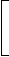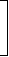# Aptitude - True Discount - Discussion

### Discussion :: True Discount - General Questions (Q.No.2)

2.

The true discount on Rs. 2562 due 4 months hence is Rs. 122. The rate percent is:

[A]. 12%
[B].
 13 1 % 3
[C]. 15%
[D]. 14%

Explanation:

P.W. = Rs. (2562 - 122) = Rs. 2440.S.I. on Rs. 2440 for 4 months is Rs. 122.Rate =100 x 122% = 15%.
 2440 x 1 3

 Rohith said: (Sep 10, 2010) I didn't undersatnd the usage of 1/3 in this solution. Can any one help me in sorting out this problem?

 Venkadesh Virudhagiri said: (Oct 17, 2010) Due is in month, so we convert into year 4/12 = 1/3.

 S.V.S Rama Chaitanya said: (Dec 3, 2010) Why should we deduct Rs 122/- from 2562/- is there any basic concept between them please explain.

 Shreya said: (Dec 30, 2010) Yup. Rama. Here formula used=. Amount=pw+tc. Pw=amount-tc.

 Karthick said: (Jan 5, 2011) Explain for pw and tc.

 Deepan said: (Mar 9, 2011) I cant understand the solution can anyone help me.

 Arjun---≫ said: (Mar 9, 2011) @Shreya Formula used by you is wrong. It is, amount=present worth(pw)+true discount(td) and hence, its comes as pw=amount-td

 Gnana said: (Mar 13, 2011) How 15% is possible, can any one help for it.

 Aishu said: (Apr 28, 2011) 100*122/2440*(1/3) 1/3 can be written as 100*122*3/2440*1 by solving this equation we get the value 15%

 Abhishek Kumar Tiwari said: (Aug 10, 2011) What is the P.W ? I know 4 month is the 1/3 of the year. But I don't no P.W.

 Yazhini said: (Aug 21, 2011) Totally there are 12 months int the year. Hence 4/12months=1/3.

 Kathya said: (Nov 2, 2011) So nice explanation.

 Rajeev said: (Dec 8, 2011) Since time and intrest must be calculated in year so month convert into year.

 Hannah said: (Dec 16, 2011) Im not able to understand by seeing the sum which formula i have to use.

 Deepa said: (Jan 17, 2012) amt=present worth + true discount pw=amt-td 2562-122 pw=2440 TIME=4MONTHS/12=1/3 SI=PTR/100 PTR=SI*100 R=(SI*100)/PT R=(122*100)/2440*1/3 R=15%

 Kumar said: (Mar 3, 2012) Are SI and td are equal. ? please explain.

 Amal Raj said: (May 2, 2012) Please explain SI.

 Mamta said: (Jul 4, 2012) What is the difference between SI and TD ? please tell me.

 Goku said: (Aug 6, 2012) Friends we can say T.D. = Interest on P.W. Hence the formula seems the same.

 Slovan said: (Nov 16, 2012) What about this? 122= 4months => 1month = 30.5 => for a year 12*30.5= 366 2562-122= 2440 366/2440= 0.15 => 15%

 Suyeb said: (Apr 21, 2013) What does true discount mean? is it means the amount of interest for a certain time of a certain amount of money?

 Thunderhemu said: (Aug 5, 2013) How come 15% I can't understand since 122 is the interest for 4 months then for each month it is 30.5, Then it should be 12.5%, Since 10% 24.4 and 2.5 % is 6.1 so the should be 12.5%. I guess can you explain it please.

 Priyanka said: (Aug 25, 2013) Rate percent is normally calculated for a year by doing 30.5/2440 we are not calculating for a year since 30.5 corresponds to a month only.

 Aachu..... said: (Oct 15, 2013) Friends, SI = interest on principal amount, in the same way, TD = interest on present worth,so v take PW instead of principal. In question they gave TD with principal,so we first calculate the PW from principal & TD using the formula PW= principal-TD and then we calculate rate using the formula which is similar to the SI.

 Dinesh Kumar Sharma said: (Dec 6, 2013) Dear guys: Don't think so much about the formula of personal wealth and discount. Moreover its conceptual question. If someone returning money in 4 month he will get discount of INR 122 over the amount. So instead of paying 2562 he is only paying = 2440 before 4 month otherwise he will pay 2562 after 4 month. So 2562-2440 = 122 is interest which we getting at the end of 4 month. So simple interest is = 122. Formula for s.i = p*r*t/100. So r = s.i*100/p*t(time should be in year so we use 1/3 year). r = 122*100/2440*1/3 = 15%.

 Ram said: (Mar 14, 2014) @Deepa you are only used in correct formula.

 Ammy said: (Apr 5, 2014) Answer explanation: PW= 100*amount/R*T. Therefore from the above formula , we need to find R. Hence formula becomes, R= 100*amount/P*T ------(1). Here amount = Rs.122 is the discount amount for 4 years. P = 2562-122 = 2418 is the original amount after applying discount. T= 4 out of 12 months i.e = 4/12 = 1/3. Substitute the values in (1), you will get the answer

 Sravanthi said: (Aug 20, 2014) Is present worth and principal amount same here? But in the previous question he calculated pw is 100 and principal is 122?

 Amit said: (Sep 9, 2014) Can anyone share formula of calculating rate of interest?

 Suhasini said: (Jan 29, 2015) Hi, I can't understand the true discount concept. Can anyone explain me clearly step by step?

 Khin Myat Kyu said: (Apr 27, 2015) 2562 is sum of the money borrowed and true discount for 4 months. So 2562 = The money borrowed+122. The money borrowed = 2562-122 = 2440. Then, 4 months (1/3 year) ===> 122. 12 months (1 year) ===> 366. And, Rs. 2440 ===> 366. Rs. 100 ===> (100*366)/2440 = 15 %

 Raman said: (Nov 11, 2015) 2562 is money = B.D = 122. 2562-122 = 2440. Then 4/12 = 1/3. 1/3 = 122 days. 1/12 = 366 days. 100*366/2440 = 15%.

 Sakthivel said: (Nov 16, 2015) P.W = Amount - T.D. So P.W = 2440. T.D = (P.W)*R*T. 100 they ask rate percent. So R = (100*T. D)/(P.W*T) %. R = (100*122)/(2440*1/3). = (100*122*3)/(2440*1). Answer: 15%.

 Divyesh said: (Feb 7, 2016) S.I means?

 Ganesh Tandel said: (Feb 25, 2016) In the question there must not mention that true discount amount is inclusive or exclusive on Rs. 2562/-. How anyone could assumed to be that? Answer should be 14% if it is exclusive or 15% if it is inclusive.

 Ankush said: (Aug 8, 2016) I don't understand the concept of true discount. Can anyone help?

 K.Jeyendran said: (Aug 13, 2016) @Ankush. Amount = present worth + true discount. pw = amt - td. 2562 - 122. pw = 2440. TIME = 4 MONTHS / 12 =1/3. SI = PTR / 100. PTR = SI * 100. R = (SI * 100) /PT. R = (122 * 100) /2440 * 1/3. You have finally get the answer for the rate of present value, below. R = 15%.

 Sahil Khann said: (Mar 11, 2017) Excellent work @Slovan.

 Purushottam said: (Jan 4, 2018) I don't understand it. Please explain me.

 Biju said: (Oct 8, 2018) I don't understand it. Can you please explain me?

 Anomies said: (Dec 4, 2018) 2562 should be present worth then why its taken as amount?

 Dashrath Kumar said: (Jul 26, 2019) Amount = present worth(pw) + true discount.

 Raghul said: (Nov 27, 2019) Easy, I'll explain: T.D =122;Amount = 2562. Hence, initial amount = 2562 - 122 = 2440. Pnr/100 = 122. 2440*4/12*x/100 = 122. Ans:x = 15%.

 Saravanan said: (Aug 31, 2021) The true discount is always reckoned on the amount. It is given in the previous formula page; So the given value is taken as amount, Now, P.W. =amount - T.D. Hope you all understood.